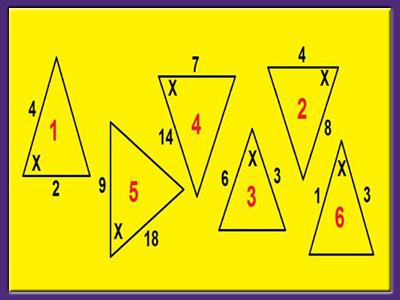All the triangles in the diagram above are similar – except one. Which triangle is the odd man out? Question 8 awaits your answer...

# Similarity (F)

If you have already tried the Congruence and Mathematical Definitions quizzes you will have a good understanding of similarity, but how well can you apply it? Test yourself in the GCSE Maths quiz!

Similarity is very close to congruence, but is slightly relaxed in that the length of corresponding sides don’t have to be equal, but they do have to have the same scale factor. Therefore, similarity is also connected with ENLARGEMENT. If we enlarge a shape, we apply a scale factor to each side. The new shape, the image, will be similar to the original shape, the object. The corresponding angles won’t have changed.

One way to look at similarity problems is to identify pairs of corresponding sides. Does each pair have the same scale factor? If so, then the two shapes are similar.

Conversely, if you are told the two shapes are similar, you can work out what the scale factor is then use it to calculate the missing lengths.

Just as with congruency, you can prove similarity in triangles by seeing if one of four rules apply:

• ASA – Angle, Side, Angle. Show that 2 angles in each triangle are the same, and you can work out the scale factor from the corresponding sides.
• SAS – Side, included Angle, Side. Show that 2 pairs of corresponding sides have the same scale factor, and the angle formed between these 2 sides is equal.
• SSS – Side, Side, Side. Show that all 3 pairs of corresponding sides have the same scale factor.
• RHS – Right-angle, Hypotenuse, Side. This is a special case for right-angled triangles. Show that the hypotenuse and one other side of each triangle have the same scale factor.

Question 1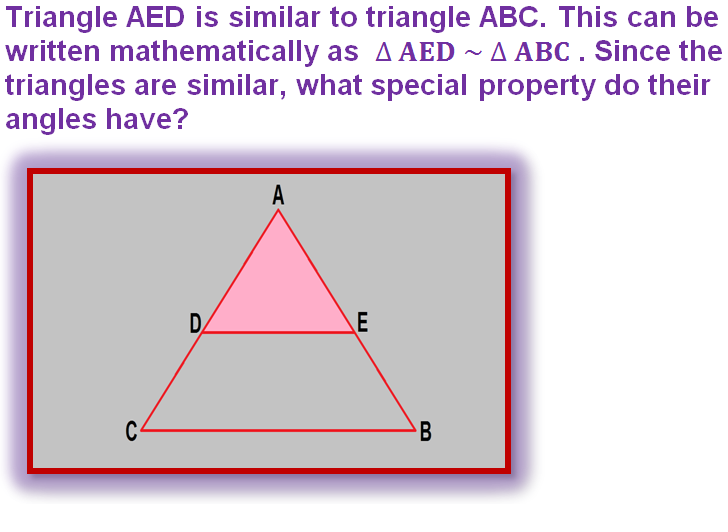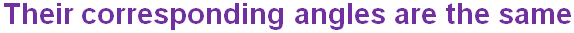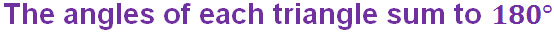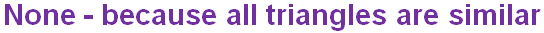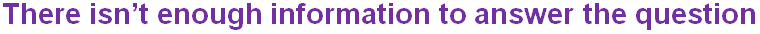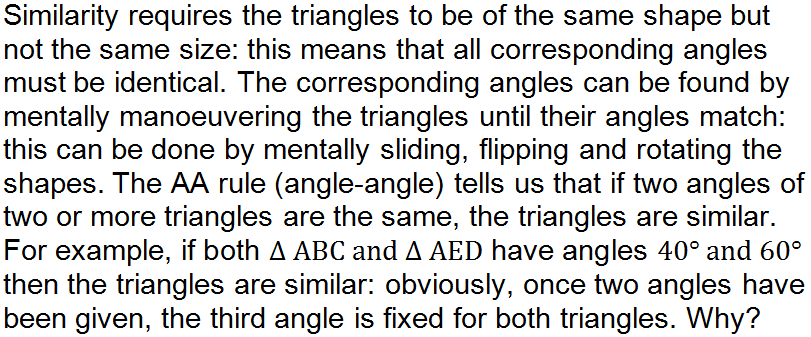Question 2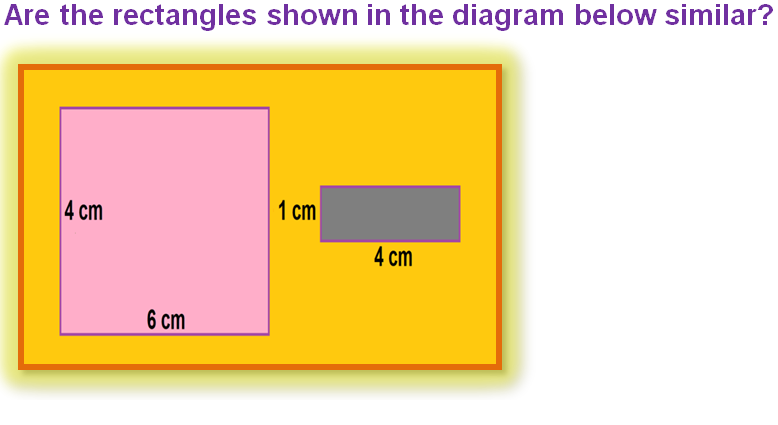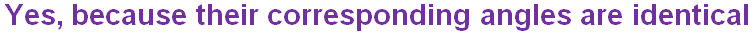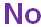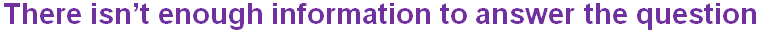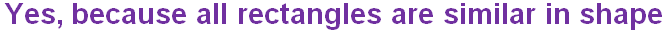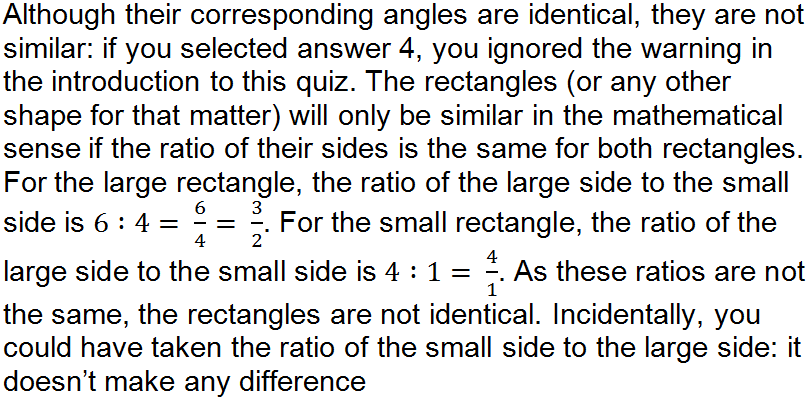Question 3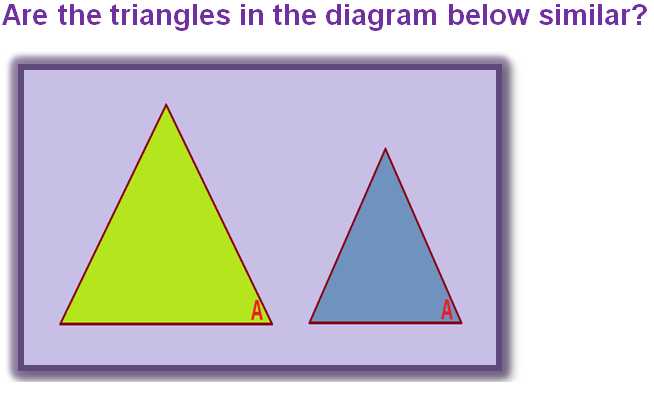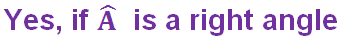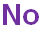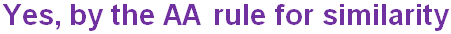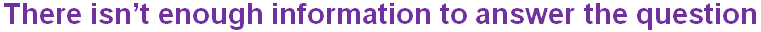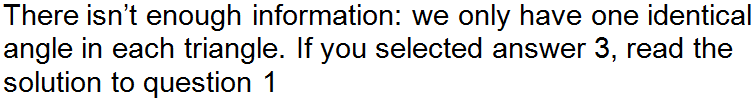Question 4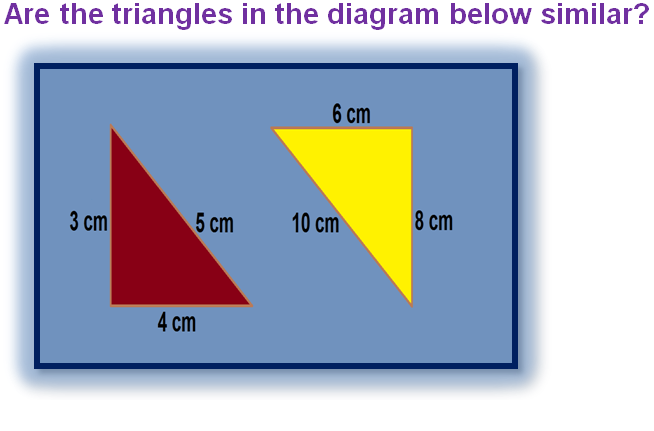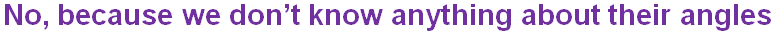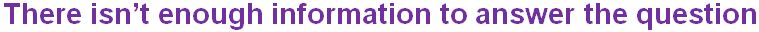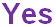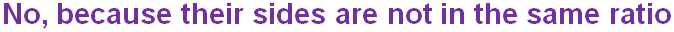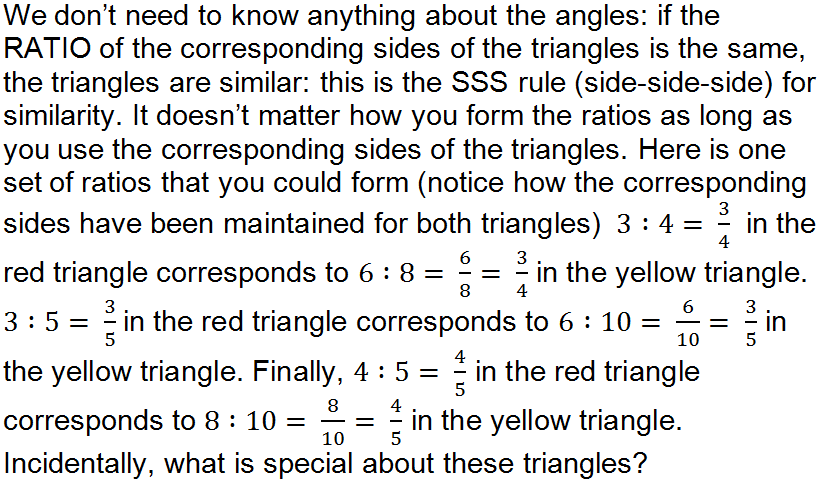Question 5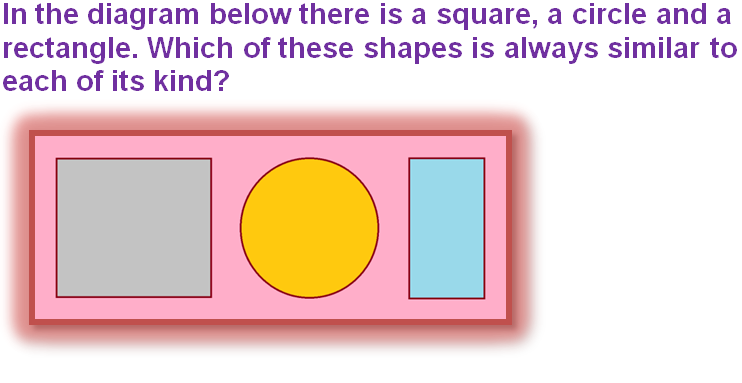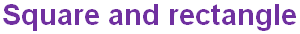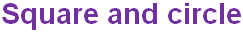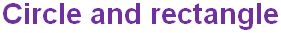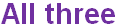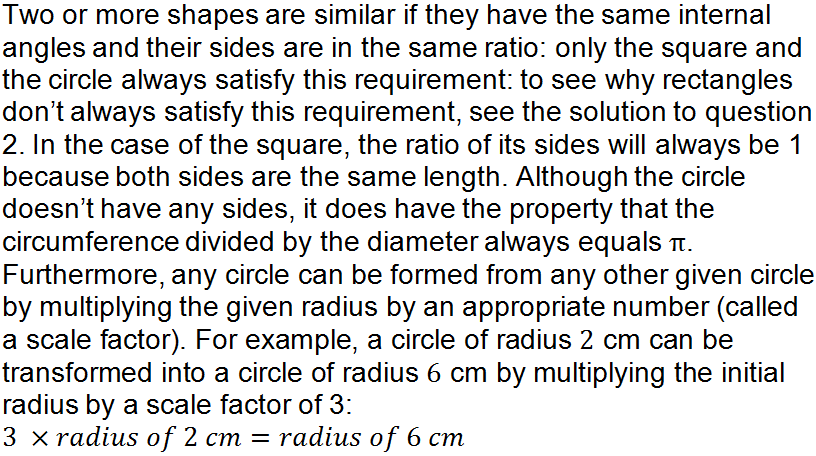Question 6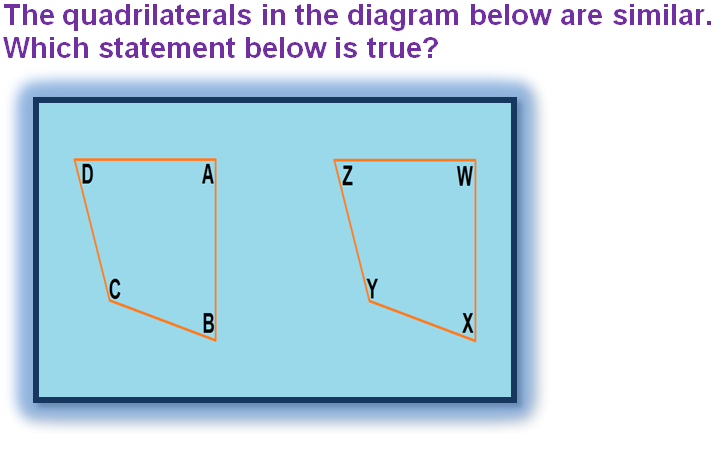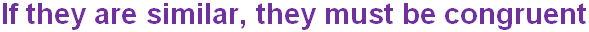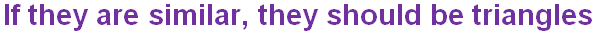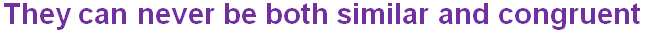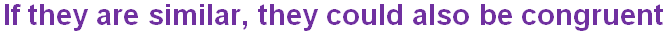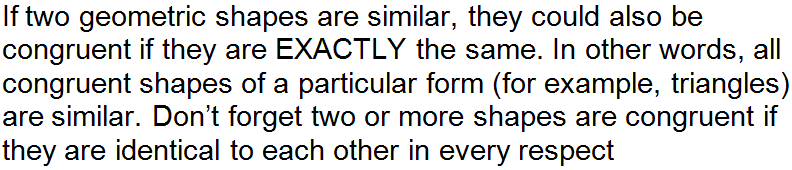Question 7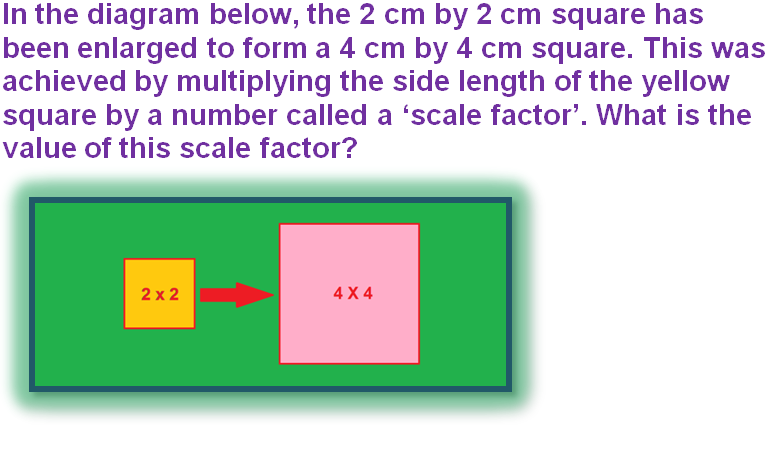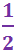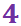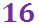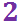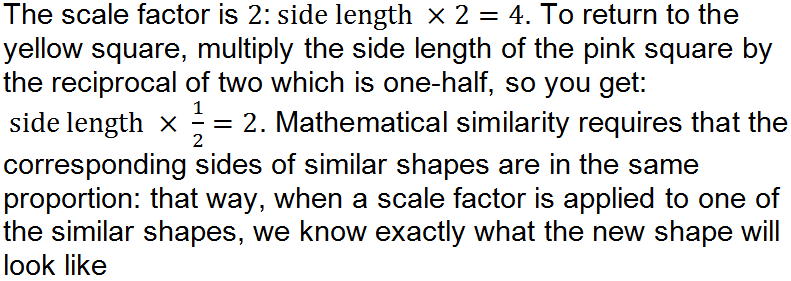Question 8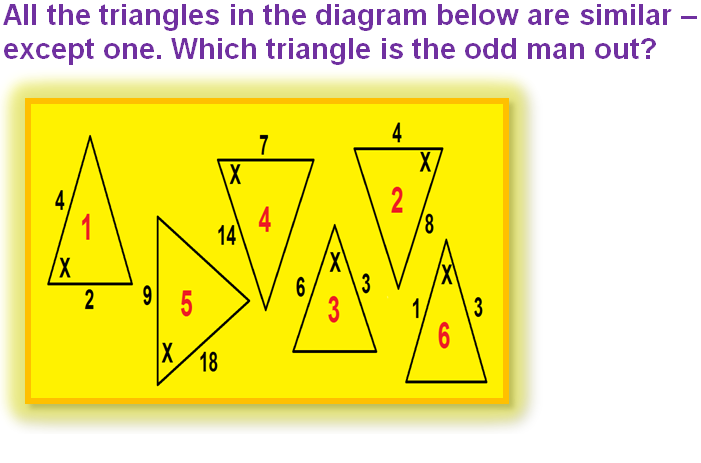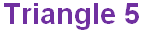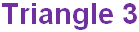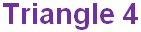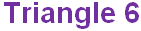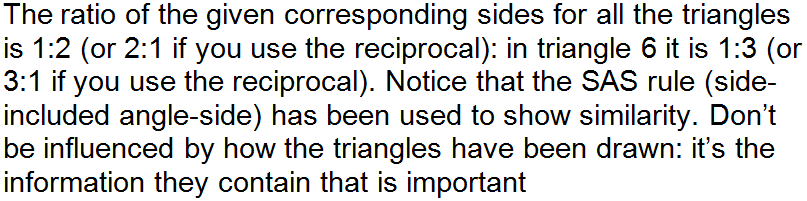Question 9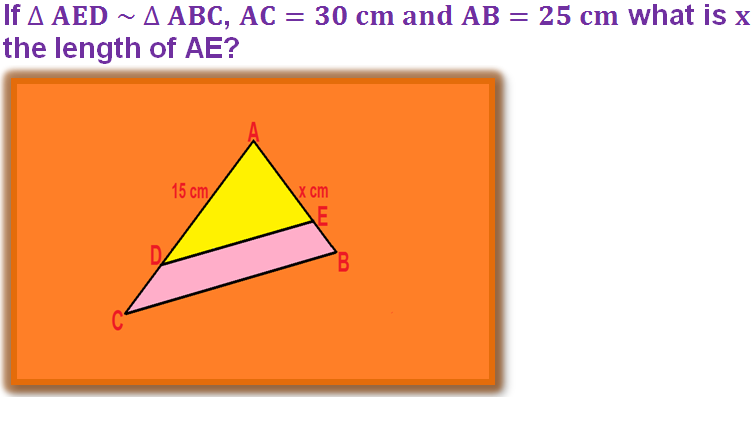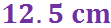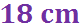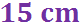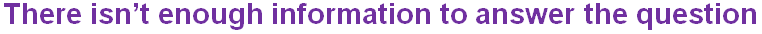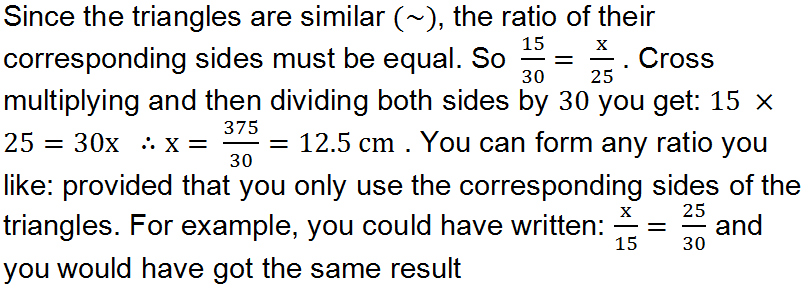Question 10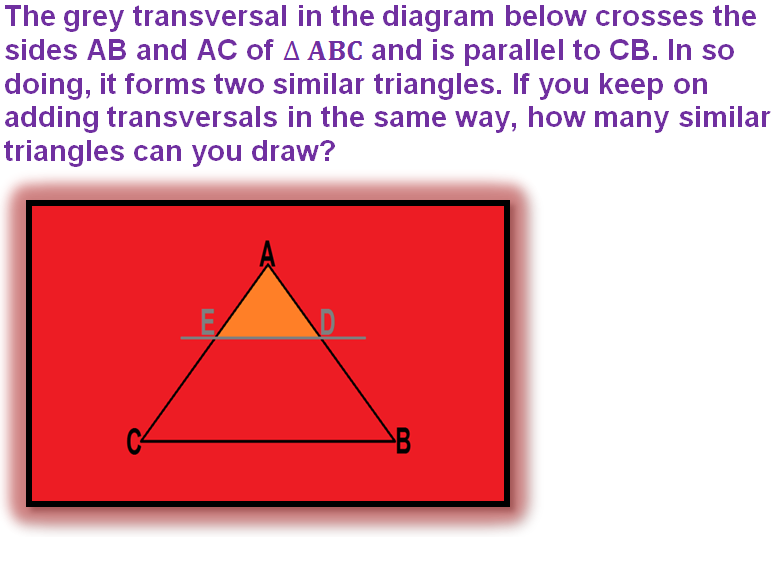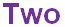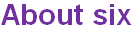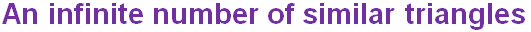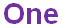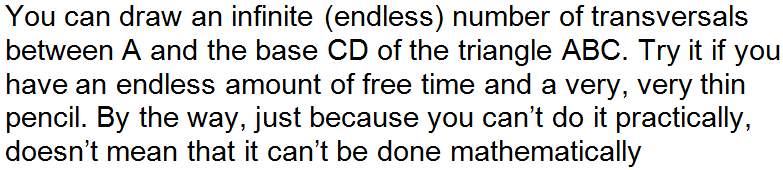Author:  Frank Evans

We use cookies to make your experience of our website better.

To comply with the new e-Privacy directive, we need to ask for your consent -